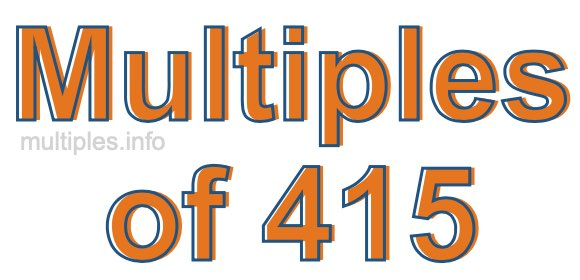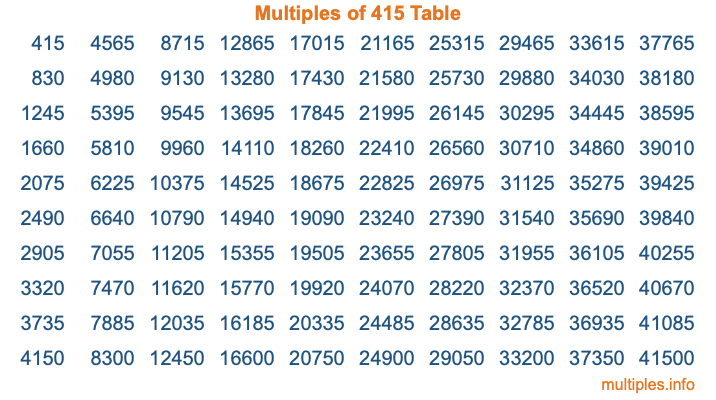Multiples of 415Welcome to the Multiples of 415 page. Here we will first teach you everything you will ever need to know about the multiples of 415, and then give you a study guide summary of everything we taught you to make sure you remember it all. Use this page to look up facts and learn information about the multiples of 415. This page will make you a multiples of four hundred fifteen expert!

Definition of Multiples of 415
Multiples of 415 are all the numbers that when divided by 415 equal an integer. Each of the multiples of 415 are called a multiple. A multiple of 415 is created by multiplying 415 by an integer.

Therefore, to create a list of multiples of 415, you start with 1 multiplied by 415, then 2 multiplied by 415, then 3 multiplied by 415, and so on for as long as you want. Thus, the list of the first five multiples of 415 is 415, 830, 1245, 1660, and 2075. To see a larger list of multiples of 415, see the printable image of Multiples of 415 further down on this page. We also have a category where you can choose any nth multiple of 415.

Multiples of 415 Checker
The Multiples of 415 Checker below checks to see if any number of your choice is a multiple of 415. In other words, it checks to see if there is any number (integer) that when multiplied by 415 will equal your number. To do that, we divide your number by 415. If the the quotient is an integer, then your number is a multiple of 415.

Is  a multiple of 415?

Least Common Multiple of 415 and ...
A Least Common Multiple (LCM) is the lowest multiple that two or more numbers have in common. This is also called the smallest common multiple or lowest common multiple and is useful to know when you are adding our subtracting fractions. Enter one or more numbers below (415 is already entered) to find the LCM.

Check out our LCM Calculator if you need more details about the Least Common Multiple or if you need the LCM for different numbers for adding and subtraction fractions.

nth Multiple of 415
As we stated above, 415 is the first multiple of 415, 830 is the second multiple of 415, 1245 is the third multiple of 415, and so on. Enter a number below to find the nth multiple of 415.

th multiple of 415

Multiples of 415 vs Factors of 415
415 is a multiple of 415 and a factor of 415, but that is where the similarities end. All postive multiples of 415 are 415 or greater than 415. All positive factors of 415 are 415 or less than 415.

Below is the beginning list of multiples of 415 and the factors of 415 so you can compare:

Multiples of 415: 415, 830, 1245, 1660, 2075, etc.

Factors of 415: 1, 5, 83, 415

As you can see, the multiples of 415 are all the numbers that you can divide by 415 to get a whole number. The factors of 415, on the other hand, are all the whole numbers that you can multiply by another whole number to get 415.

It's also interesting to note that if a number (x) is a factor of 415, then 415 will also be a multiple of that number (x).

Multiples of 415 vs Divisors of 415
The divisors of 415 are all the integers that 415 can be divided by evenly. Below is a list of the divisors of 415.

Divisors of 415: 1, 5, 83, 415

The interesting thing to note here is that if you take any multiple of 415 and divide it by a divisor of 415, you will see that the quotient is an integer.

Multiples of 415 Table
Below is an image of the first 100 multiples of 415 in a table. The table is in chronological order, column by column. The first column has the first ten multiples of 415, the second column has the next ten multiples of 415, and so on.The Multiples of 415 Table is also referred to as the 415 Times Table or Times Table of 415. You are welcome to print out our table for your studies.

Negative Multiples of 415
Although not often discussed or needed in math, it is worth mentioning that you can make a list of negative multiples of 415 by multiplying 415 by -1, then by -2, then by -3, and so on, to get the following list of negative multiples of 415:

-415, -830, -1245, -1660, -2075, etc.

Multiples of 415 Summary
Below is a summary of important Multiples of 415 facts that we have discussed on this page. To retain the knowledge on this page, we recommend that you read through the summary and explain to yourself or a study partner why they hold true.

There are an infinite number of multiples of 415.

A multiple of 415 divided by 415 will equal a whole number.

415 divided by a factor of 415 equals a divisor of 415.

The nth multiple of 415 is n times 415.

The largest factor of 415 is equal to the first positive multiple of 415.

415 is a multiple of every factor of 415.

415 is a multiple of 415.

A multiple of 415 divided by a divisor of 415 equals an integer.

415 divided by a divisor of 415 equals a factor of 415.

Any integer times 415 will equal a multiple of 415.

Multiples of a Number
Here you can get the multiples of another number, all with the same attention to detail as we did for multiples of 415 on this page.

Multiples of
Multiples of 416
Did you find our page about multiples of four hundred fifteen educational? Do you want more knowledge? Check out the multiples of the next number on our list!